# Excel SUMPRODUCT function with formula examples

The tutorial explains the basic and advanced uses of the SUMPRODUCT function in Excel. You will find a number of formula examples to compare arrays, conditionally sum and count cells with multiple criteria, calculate a weighted average and more.

When you hear the name of SUMPRODUCT for the first time, it may sound like some useless formula that performs an ordinary sum of the products operation. But that definition does not show even a tiny fraction of what Excel SUMPRODUCT is capable of.

In fact, SUMPRODUCT is a remarkably versatile function with many uses. Due to its unique ability to handle arrays in smart and elegant ways, SUMPRODUCT is extremely useful, if not indispensable, when it comes to comparing data in two or more ranges and calculating data with multiple criteria. The following examples will reveal the full power of SUMPRODUCT and its effectiveness will become crystal clear.

## Excel SUMPRODUCT function - syntax and uses

Technically, the SUMPRODUCT function in Excel multiplies the numbers in the specified arrays, and returns the sum of those products.

The syntax of the SUMPRODUCT function is simple and straightforward:

SUMPRODUCT(array1, [array2], [array3], …)

Where array1, array2, etc. are continuous ranges of cells or arrays whose elements you want to multiply, and then add.

The minimum number of arrays is 1. In this case, a SUMPRODUCT formula simply adds up all of the array elements and returns the sum.

The maximum number of arrays is 255 in Excel 2016, Excel 2013, Excel 2010, and Excel 2007, and 30 in earlier Excel versions.

Although SUMPRODUCT works with arrays, it does not require using the array shortcut (Ctrl + Shift + Enter). You compete a SUMPRODUCT formula in a usual way by pressing the Enter key.

Notes:

• All arrays in a SUMPRODUCT formula must have the same number of rows and columns, otherwise you get the #VALUE! error.
• If any array argument contains non-numeric values, they will be treated as zeros.
• If an array is a logical test, it results in TRUE and FALSE values. In most cases, you'd need to convert them to 1 and 0 by using the double unary operator (--) . Please see the SUMPRODUCT with multiple criteria example for more details.
• SUMPRODUCT does not support wildcard characters.

### Basic usage of SUMPRODUCT in Excel

To gain a general understanding of how the Excel SUMPRODUCT function works, consider the following example.

Supposing you have quantity in cells A2:A4, prices in cells B2:B4, and you wish to find out the total. If you were doing a school math test, you would multiply the quantity by price for each item, and then add up the subtotals. In Microsoft Excel, you can get the result with a single SUMPRODUCT formula:

`=SUMPRODUCT(A2:A4,B2:B4)`

The following screenshots shows it in action: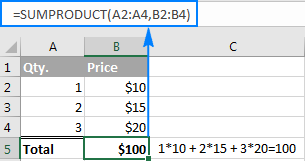Here is what's going on under the hood in terms of math:

• The formula takes the 1st number in the 1st array and multiplies it by the 1st number in the 2nd array, then takes the 2nd number in the 1st array and multiplies it by the 2nd number in the 2nd array, and so on.
• When all of the array elements are multiplied, the formula adds up the products and returns the sum.

In other words, our SUMPRODUCT formula performs the following mathematical operations:

`=A2*B2 + A3*B3 + A4*B4`

Just think how much time it could save you if your table contained not 3 rows of data, but 3 hundred or 3 thousand rows!

## How to use SUMPRODUCT in Excel - formula examples

Multiplying two or more ranges together and then summing the products is the simplest and most obvious usage of SUBTOTAL in Excel, though not by far the only one. The real beauty of the Excel SUMPRODUCT function is that it can do far more than its stated purpose. Further on in this tutorial, you will find a handful of formulas that demonstrate more advanced and exciting uses, so please keep reading.

### SUMPRODUCT with multiple criteria

Usually in Microsoft Excel, there is more than one way to accomplish the same task. But when it comes to comparing two or more arrays, especially with multiple criteria, SUMPRODUCT is the most effective, if not the only, solution. Well, either SUMPRODUCT or array formula.

Assuming you have a list of items in column A, planned sale figures in column B, and actual sales in column C. Your goal is to find out how many items have made less sales than planned. For this, use one of the following variations of the SUMPRODUCT formula:

`=SUMPRODUCT(--(C2:C10<B2:B10))`

or

`=SUMPRODUCT((C2:C10<B2:B10)*1)`

Where C2:C10 are real sales and B2:B10 are planned sales.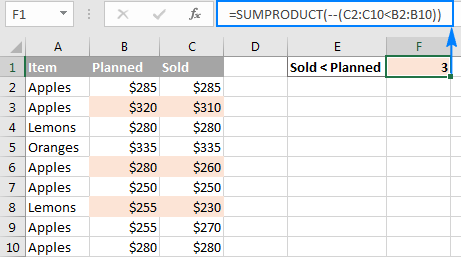But what if you had more than one condition? Let's say, you want to count how many times Apples performed worse than planned. The solution is to add one more criterion to the SUMPRODUCT formula:

`=SUMPRODUCT(--(C2:C10<B2:B10), --(A2:A10="apples"))`

Or, you can use the following syntax:

`=SUMPRODUCT((C2:C10<B2:B10)*(A2:A10="apples"))`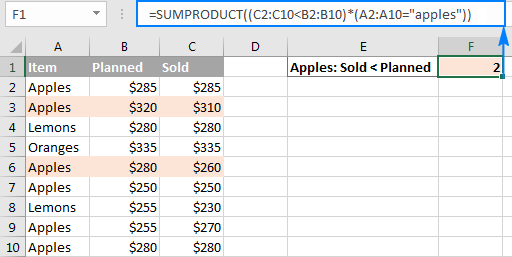And now, let's take a minute and understand what the above formulas are actually doing. I believe it is a worthy time investment because many other SUMPRODUCT formulas work with the same logic.

#### How SUMPRODUCT formula with one condition works

For starters, let's break down a simpler formula that compares numbers in 2 columns row-by-row, and tells us how many times column C is less than column B:

`=SUMPRODUCT(--(C2:C10<B2:B10))`

If you select the portion (C2:C10<B2:B10) in the formula bar, and press F9 to view the underlying values, you will see the following array: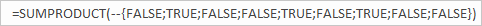What we have here is an array of Boolean values TRUE and FALSE, where TRUE means the specified condition is met (i.e. a value in column C is less than a value in column B in the same row), and FALSE signifies the condition is not met.

The double negative (--), which is technically called the double unary operator, coerces TRUE and FALSE into ones and zeros: {0;1;0;0;1;0;1;0;0}.

Another way to convert the logical values into the numeric values is multiple the array by 1:

`=SUMPRODUCT((C2:C10<B2:B10)*1)`

Either way, since there is just one array in the SUMPRODUCT formula, it simply adds up 1's in the resulting array and we get the desired count. Easy, isn't it?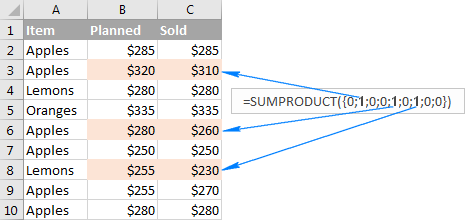#### How SUMPRODUCT formula with multiple conditions works

When an Excel SUMPRODUCT formula contains two or more arrays, it multiplies the elements of all the arrays, and then adds up the results.

As you may remember, we used the following formulas to find out how many times the number of real sales (column C) was less than planned sales (column B) for Apples (column A):

`=SUMPRODUCT(--(C2:C10<B2:B10), --(A2:A10="apples"))`

or

`=SUMPRODUCT((C2:C10<B2:B10)*(A2:A10="apples"))`

The only tech difference between the formulas is the method of coercing TRUE and FALSE into 1 and 0 - by using the double unary or multiplication operation. As the result, we get two arrays of ones and zeros: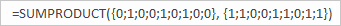The multiplication operation performed by SUMPRODUCT joins them into a single array. And since multiplying by zero always gives zero, 1 appears only when both conditions are met, and consequently only those rows are counted: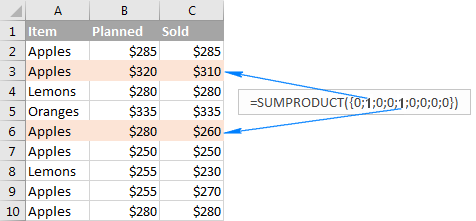### Conditionally count / sum / average cells with multiple criteria

In Excel 2003 and older versions that did not have the so-called IFs functions, one of the most common uses of the SUMPRODUCT function was to conditionally sum or count cells with multiple criteria. Beginning with Excel 2007, Microsoft introduced a series of functions specially designed for such tasks - SUMIFS, COUNTIFS and AVERAGEIFS.

But even in the modern versions of Excel, a SUMPRODUCT formula could be a worthy alternative, for example, to conditionally sum and count cells with the OR logic. Below you will find a few formula examples that demonstrate this ability in action.

#### 1. SUMPRODUCT formula with AND logic

Supposing you have the following dataset, where column A lists the regions, column B - items and column C - sales figures: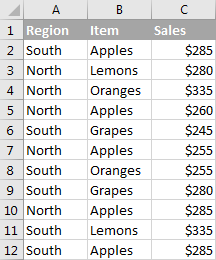What you want is get the count, sum and average of Apples sales for the North region.

In Excel 2007 and higher, the task can be easily accomplished by using a SUMIFS, COUNTIFS and AVERAGEIFS formula. If you are not looking for easy ways, or if you are still using Excel 2003 or older, you can get the desired result with SUMPRODUCT.

• To count Apples sales for North:`=SUMPRODUCT(--(A2:A12="north"), --(B2:B12="apples"))`

or

`=SUMPRODUCT((A2:A12="north")*(B2:B12="apples"))`

• To sum Apples sales for North:`=SUMPRODUCT(--(A2:A12="north"), --(B2:B12="apples"), C2:C12)`

or

`=SUMPRODUCT((A2:A12="north")*(B2:B12="apples")*C2:C12)`

• To average Apples sales for North:To calculate the average, we simply divide Sum by Count like this:

`=SUMPRODUCT(--(A2:A12="north"), --(B2:B12="apples"), C2:C12) / SUMPRODUCT( --(A2:A12="north"), --(B2:B12="apples"))`

To add more flexibility to your SUMPRODUCT formulas, you can specify the desired Region and Item in separate cells, and then reference those cells in your formula like shown in the screenshot below: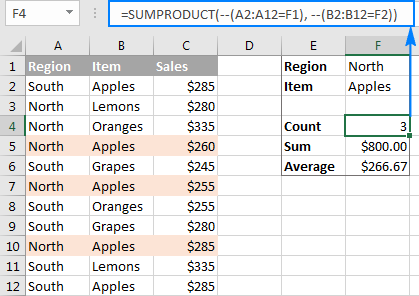How SUMPRODUCT formula for conditional sum works

From the previous example, you already know how the Excel SUMPRODUCT formula counts cells with multiple conditions. If you understand that, it will be very easy for you to comprehend the sum logic.

Let me remind you that we used the following formula to sum Apples sales in the North region:

`=SUMPRODUCT(--(A2:A12="north"), --(B2:B12="apples"), C2:C12)`

An intermediate result of the above formula are the following 3 arrays: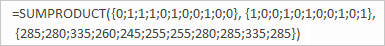• In the 1st array, 1 stands for North, and 0 for any other region.
• In the 2nd array, 1 stands for Apples, and 0 for any other item.
• The 3rd array contains the sales numbers exactly as they appear in cells C2:C12.

Remembering that multiplying by 0 always gives zero, and multiplying by 1 gives the same number, we get the final array consisting of the sales numbers and zeros - a sales number appears only if the first two arrays have 1 in the same position, i.e. both of the specified conditions are met; zero otherwise: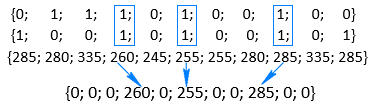Adding up the numbers in the above array delivers the desired result - the total of the Apples sales in the North region.

#### Example 2. SUMPRODUCT formula with OR logic

To conditionally sum or count cells with the OR logic, use the plus symbol (+) in between the arrays.

In Excel SUMPRODUCT formulas, as well as in array formulas, the plus symbol acts like the OR operator that instructs Excel to return TRUE if ANY of the conditions in a given expression evaluates to TRUE.

For example, to get the count of all Apples and Lemons sales regardless of the region, use this formula:

`=SUMPRODUCT((B2:B12="apples")+(B2:B12="lemons"))`

Translated into plain English, the formula reads as follows: Count cells if B2:B12="apples" OR B2:B12="lemons".

To sum Apples and Lemons sales, add one more argument containing the Sales range:

`=SUMPRODUCT((B2:B12="apples")+(B2:B12="lemons"), C2:C12)`

The following screenshot shows a similar formula in action: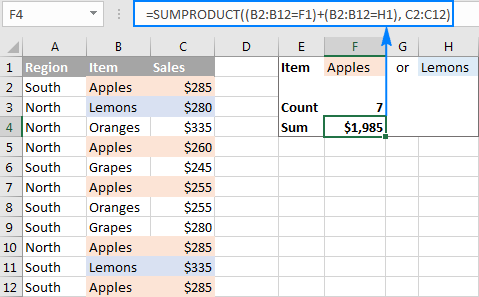#### Example 3. SUMPRODUCT formula with AND as well as OR logic

In many situations, you might need to conditionally count or sum cells with AND logic and OR logic at a time. Even in the latest versions of Excel, the IFs series of functions is not capable of that.

One of the possible solutions is combining two or more functions SUMIFS + SUMIFS or COUNTIFS + COUNTIFS.

Another way is using the Excel SUMPRODUCT function where:

• Asterisk (*) is used as the AND operator.
• Plus symbol (+) is used as the OR operator.

To make things easier to understand, consider the following examples.

To count how many times Apples and Lemons were sold in the North region, make a formula with the following logic:

`=Count If ((Region="north") AND ((Item="Apples") OR (Item="Lemons")))`

Upon applying the appropriate SUMPRODUCT syntax, the formula takes the following shape:

`=SUMPRODUCT((A2:A12="north")*((B2:B12="apples")+(B2:B12="lemons")))`

To sum Apples and Lemons sales in the North region, take the above formula and add the Sales array with the AND logic:

`=SUMPRODUCT((A2:A12="north")*((B2:B12="apples")+(B2:B12="lemons"))*C2:C12)`

To make the formulas a bit more compact, you can type the variables in separate cells - Region in F1 and Items in F2 and H2 - and refer to those cells in your formula: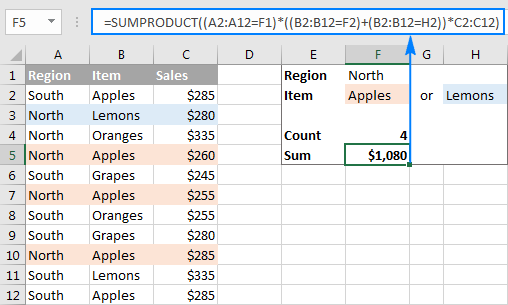### SUMPRODUCT formula for weighted average

In one of the previous examples, we discussed a SUMPRODUCT formula for conditional average. Another common usage of SUMPRODUCT in Excel is calculating a weighted average where each value is assigned a certain weight.

The generic SUMPRODUCT weighted average formula is as follows:

SUMPRODUCT(values, weights) / SUM(weights)

Assuming that values are in cells B2:B7 and weights are in cell C2:C7, the weighted average SUMPRODUCT formula will look like this:

`=SUMPRODUCT(B2:B7,C2:C7)/SUM(C2:C7)`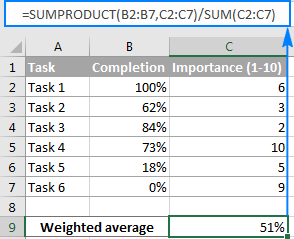I believe at this point you won't have any difficulties with understanding the formula logic. If someone needs a detailed explanation, please check out the following tutorial: Calculating weighted average in Excel.

### SUMPRODUCT as alternative to array formulas

Even if you are reading this article for informational purposes and the details are likely to fade away in your memory, remember just one key point - the Excel SUMPRODUCT function deals with arrays. And because SUMPRODUCT offers much of the power of array formulas, it can become an easy-to-use replacement for them.

What advantages does this gives to you? Basically, you will be able to manage your formulas an easy way without having to press Ctrl + Shift + Enter every time you are entering a new or editing an existing array formula.

As an example, we can take a simple array formula that counts all characters in a given range:

{=SUM(LEN(range))}

and turn it into a regular formula:

=SUMPRODUCT(LEN(range))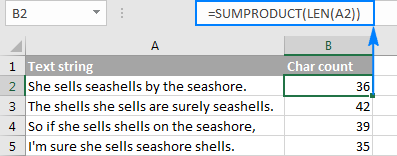For practice, you can take these Excel array formulas and try to re-write then using the SUMPRODUCT function.

### Excel SUMPRODUCT - advanced formula examples

Now that you know the syntax and logic of the SUMPRODUCT function in Excel, you may want to learn more sophisticated and more powerful formulas where SUMPRODUCT is used in liaison with other Excel functions.